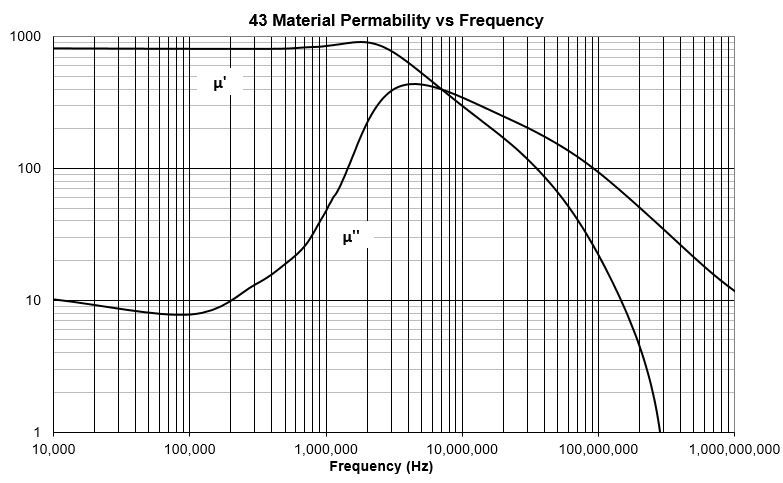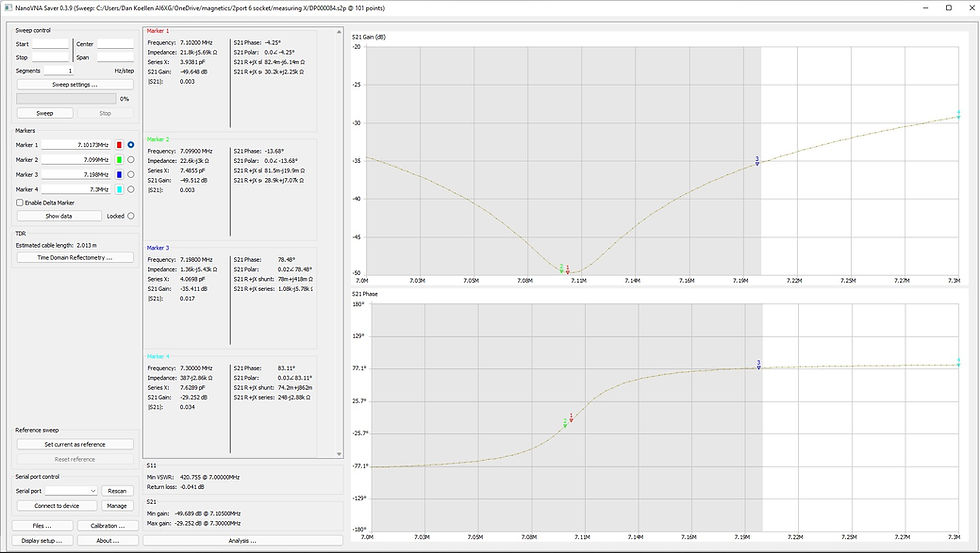top of page
• Dan Koellen AI6XG

# Reactive Component Measurement With the NanoVNA

Updated: Aug 15, 2022

Recently I was experimenting with output networks for a portable QRP tube transmitter and wanted to measure the inductances used at the frequency of use. This post will summarize information I found about measurements of inductors and capacitors, present some data over various values of impedance using the nanoVNA and summarize my observations.

## Measuring Inductance and Capacitance

There are instruments available to measure inductance and capacitance but many measure at a specific or a small band of frequencies. But reactive components may have their value dependent on frequency as well as impedance that varies by frequency. For example, a ferrite core inductor's permeability is complex and dependent on frequency. So measuring an inductor wound on a ferrite core at one frequency will only suffice if you are using the inductor at that frequency.Figure 1

There are many references (see appendix) available that discuss measuring impedance (i.e. inductance or capacitance) with a Vector Network Analyzer (VNA). Much of the discussion is made using measurements from laboratory grade VNAs with true two port measurements. In-depth analysis of errors was also made but beyond the scope of this discussion. But we can use the basic setups when working with the nanoVNA, albeit the range of impedances that may be measured and the accuracy will not be the same as a laboratory grade VNA. As we will see, especially considering its price point, the nanoVNA is quite capable.

## Measurement Configurations

There are three configurations for impedance measurements using a two port VNA. They are the shunt, shunt through and series (also referred to as series through) configurations shown below:Figure 2 Three Configurations for Impedance Measurements (Reference 1)

The shunt configuration is very easy to use with a one or two port VNA, simply solder the device under test (DUT) across a connector that mates with the VNA port. The other two configurations require a test fixture of some sort. I built a simple text fixture with convenient header sockets so DUTs and the configuration could be changed easily. The SMA connectors were spaced so they directly connect to the nanoVNA port connectors

Figure 3 Test Fixture

According to the literature, each configuration has a specific range of impedances that they are optimum for. To explore this, let's first look at how to derive impedance from the S parameters that are obtained from each configuration measurement (Z0 is the characteristic impedance of the VNA port, usually 50 Ohms).

Z = Z0*(1 + S11)/(1 - S11) Shunt Equation 1

Z = Z0*S21/(2*(1 - S21)) Shunt Through Equation 2

Z = 2*Z0*(1 - S21)/S21 Series Equation 3

Remember that the above variables and constant are complex numbers, use the appropriate arithmetic for complex numbers. The reactance of the DUT is the imaginary portion of Z, you then calculate L or C from the reactance. Below is the relationship of absolute impedance (|Z|) and S parameters over a range of impedances that are much less and greater than Z0 = 50 Ohms.The best measurements will be where there is a reasonable slope so a change in S parameters corresponds to a change in |Z|. For example, the slope of the shunt through curve provides for measurements below a few hundred Ohms, conversely for larger impedances there is little to no change in S21 for a substantial change in |Z|. From this graph the series configuration would be used for high impedances, the shunt through configuration for low impedances and the shunt configuration for impedances around Z0. To test this I measured a range of reactive components from ~10 Ohms to ~ 3k Ohms.

## Reactive Components: A Few Measurements

I used the test fixture (figure 3) described earlier to measure a range of inductors and capacitors, using the three configurations in a frequency range of 6 MHz to 7 MHz for most measurements. A capacitor and inductor were paired so their impedances matched in this frequency range, giving an additional measurement of parallel resonance where S21 gain is minimum and S21 phase = 0.

The inductors were wound on -2 powdered iron core toroids so there was minimal change in inductance with frequency. The lone exception was for high impedance measurement which I used a series combination of commercially available inductors that used ferrite core material, these coils were characterized for use in the test frequency range by the manufacturer. Silver mica and C0G ceramic capacitors were used for these measurements, their characteristics provided for good operation in the frequency band that was measured. The parallel resonant measurements showed that the both the inductors and capacitors were of good RF quality. A capacitor, of unknown material, that was to be used for extremely low impedance measurements was rejected because it was of inferior RF quality, showing poor resonance. This impedance point was not measured.

The value of the test capacitor was measured using a capacitor meter that is calibrated to a known 1 nF capacitor, the capacitance of the fixture and leads was also compensated. Since the capacitance measurement is made at a lower frequency than the text frequency range, the absolute value may not be exact for the text frequency band. But all measurements were made the same way so it will provide a means of comparison.

The measurement error was calculated in two ways. For capacitors the nanoVNA measured value was compared to the capacitor meter measured value. For inductors it is a bit more complicated. The nanoVNA measured inductance at the parallel resonance frequency was used to calculate the equivalent parallel capacitance, this equivalent capacitance was compared to the capacitor meter measured value of the actual capacitor used for resonance.

Before measurements were recorded, the nanoVNA idled for about 15 minutes to stabilize the operating temperature. A full calibration was run before each set of measurements across the measurement frequency band. A nanoVNA-F, manufactured by BH5HNU, hw version 3.1 using fw version 0.2.1 was used for the measurements.Figure 4 Summary of Impedance/Reactance Measurements. Yellow > 10%, Red > 20% Error, n/a is nonsensical data.

Figure 4 shows the summary of the data from each of the impedance measurement configurations for inductors (L) and capacitors (C) in three ranges of impedance. The data shows that series configuration for low impedances does not provide a good result. Likewise, the high impedance data shows that shunt through is not appropriate. The series measurements varied so much for the low impedance capacitance measurements on successive measurements that they were deemed nonsensical. Likewise the shunt through measurements varied greatly between successive measurements for high impedance. Surprisingly, shunt measurements provide decent data for much of the impedance range.

There are two data points that deviated from expectation. The C series measurement for 3.2k Ohm |Z| is higher than expected. For a series measurement, the test fixture the path is longer than for shunt measurements. Since the capacitor used for this impedance was 10 pF, a few pF of extra capacitance will induce a larger than expected error. A test fixture built for minimum parasitic capacitance rather than easy configuration change would likely provide a better result. For the other data point in question, the shunt through measurement showed higher than expected error for low impedance capacitor measurement. I do not have an explanation for that data point.

## Conclusions

The nanoVNA provides reasonable reactance measurements for most of the needs of a radio amateur. In cases where better accuracy and precision is required, a well calibrated laboratory VNA should be used with an appropriate test fixture.

• Avoid series measurements for low impedance.

• Avoid shunt through measurements for high impedance.

• The shunt configuration gives good results over a fairly wide range of impedances; this configuration is easy to use and calibrate, and satisfies most measurement needs.

• Proper calibration of the nanoVNA over the measurement frequency range is important.

• Suggest that the nanoVNA be turned on and let idle for a while to settle to operating temperature.

• Test fixture configuration is important, especially for small values of reactance.

• Successive measurement runs for a configuration not appropriate for the impedance being measured will show a wide variation. Likewise, an appropriate configuration will show consistent data between measurement runs.

## Data ExamplesFigure 5 Calculated Data From 25 turns T80-2 Inductor MeasurementsFigure 6 Calculated Data From 100pF plus 33pF Capacitor MeasurementsFigure 7 Parallel Resonance for |Z| 170 Ohm Reactive Components

## Appendix

1. Make Accurate Impedance Measurements Using a VNA Microwaves and RF (note that the series equation is not correct)

2. Determination of Permeability From Impedance Measurement Using Vector Network Analyzer, Rastislav Dosoudil, Journal of Electrical Engineering, vol. 63, no 7s, 2012, 97-101

3. Measuring Capacitor Parameters Using Vector Network Analyzers, Deniss Stepins et al, Electronics, vol. 18, no. 1, June 2014

4. Measuring Impedances of DC biased Inductors by Using Vector Network Analyzers, g. Asmanis et al. Intl Journal of Electronics and Telecommunications, 2019, vol 65, no. 3, pp 375-380

5. Advanced Impedance Measurement Capability of the RF I-V Method Compared to the Network Analysis Method, Agilent Application Note 1369-2